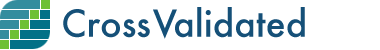## Questions and Answers per Month

0

(click on this box to dismiss)Q&A for people interested in statistics, machine learning, data analysis, data mining, and data visualization

``````select [Month],
cast(cast(sum(case when Score>=0 and AnswerCount > 0 then 1 else 0 end) as float)/
cast(sum(case when Score>=0 then 1 else 0 end) as float) as Decimal(6,3)) ratio0,
cast(cast(sum(case when Score>=1 and AnswerCount > 0 then 1 else 0 end) as float)/
cast(sum(case when Score>=1 then 1 else 0 end) as float) as Decimal(6,3)) ratio1,
cast(cast(sum(case when Score>=2 and AnswerCount > 0 then 1 else 0 end) as float)/
cast(sum(case when Score>=2 then 1 else 0 end) as float) as Decimal(6,3)) ratio2,
cast(cast(sum(case when Score>=3 and AnswerCount > 0 then 1 else 0 end) as float)/
cast(sum(case when Score>=3 then 1 else 0 end) as float) as Decimal(6,3)) ratio3,
cast(cast(sum(case when Score>=4 and AnswerCount > 0 then 1 else 0 end) as float)/
cast(sum(case when Score>=4 then 1 else 0 end) as float) as Decimal(6,3)) ratio4,
cast(cast(sum(case when Score>=4 and AnswerCount > 0 then 1 else 0 end) as float)/
cast(sum(case when Score>=4 then 1 else 0 end) as float) as Decimal(6,3)) ratio5
from
(
select CAST(
cast(DATEPART(YYYY, CreationDate) as varchar) + '-' + cast(DATEPART(MM, CreationDate) as varchar) + '-01'
as datetime) [Month],

from Posts p
where ClosedDate is NULL
and PostTypeId = 1
) as X
group by [Month]
order by [Month] asc``````

### Enter Parameters

Options:
-Hold tight while we fetch your results
:records returned in :time ms:cached